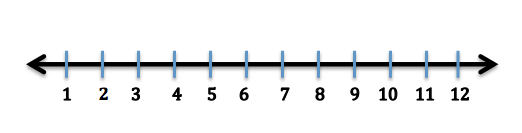# Using a Number Line for Addition & Subtraction

Instructions:

question 1 of 3

### A number line can be used to for addition by moving (jumping) _____.

Create Your Account To Take This Quiz

As a member, you'll also get unlimited access to over 79,000 lessons in math, English, science, history, and more. Plus, get practice tests, quizzes, and personalized coaching to help you succeed.

Try it risk-free for 30 days. Cancel anytime

### 2. Use the number line pictured to solve the following problem: Jeff went fishing and caught 5 silver fish and 4 brown fish. How many fish did he catch in all?Create your account to access this entire worksheet
Quizzes, practice exams & worksheets
Certificate of Completion
Create an account to get started

On this quiz and worksheet combination, you will find questions about subtracting and adding on a number line. You can see if you know how to move on a number line, and you will also be asked to perform both operations on some practice problems.

## Quiz & Worksheet Goals

Expect the following subjects to appear on this combination quiz and worksheet:

• Addition on a number line
• Subtraction on a number line
• Using a number line to solve word problems

## Skills Practiced

• Information recall - remember what you have studied about addition and subtraction
• Problem solving - use a number line to practice with word problems
• Making connections - understand how adding and subtracting are performed on number lines

For more practicing, check out the lesson titled Using a Number Line for Addition & Subtraction. There, you will see the following:

• A practice problem involving selling candy bars
• Order on a number line
• Examples of subtraction and addition
Final ExamMath for Kids
Status: Not Started
Chapter ExamNumbers for Elementary School
Status: Not Started

Support